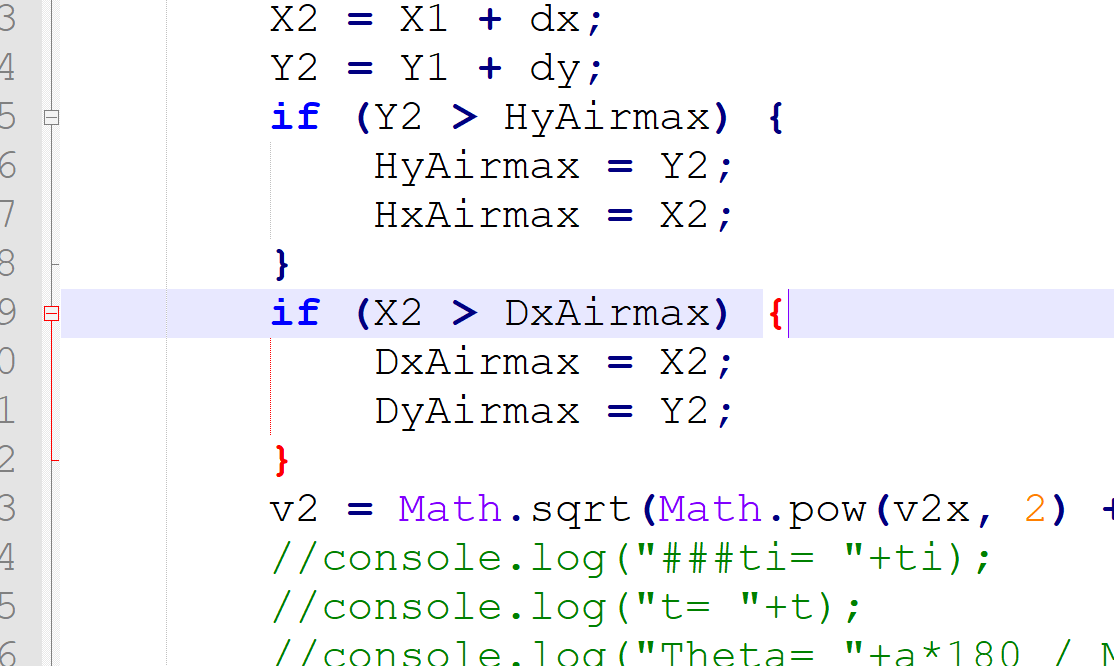# JavaScript problem

Elias Y. Daoud shared this question 2 years ago

When I click on "Undo" (my custom button). The following goes in the process. And anything written as For(...){ ...} give me the error

"} bracket is missing" refer to the attachment

var pointsNb=ggbApplet.getValue("pointsNb");

for(var ti=0;ti<=pointsNb;ti++)

{

ggbApplet.evalCommand("DeleteObject(P"+String(ti)+")");

}

too, even if I make a simple one

...

for(var ti=0;ti<=pointsNb;ti++)

{

ti=ti+1;

}

Note: this configuration or similar loop I made in another button, no error is generated.

Something illogical.

look to this:

var pointsNb=ggbApplet.getValue("pointsNb");

for (var ti=0;ti<=pointsNb;ti++)

{

v2x=(-k*v1x*Math.sqrt(Math.pow(v1x,2)+Math.pow(v1y,2)))*dt+v1x;

v2y=(-k*v1y*Math.sqrt(Math.pow(v1x,2)+Math.pow(v1y,2))-g)*dt+v1y;

....

...

}

It works properly.111

Here it is1

These two lines are swapped. Stick with ggbApplet then it will be clearer

```ggb.setUndoPoint();

var ggb = ggbApplet;```1

I am not using setUndoPoint() I put before // as a comment because it is not working or I don't know-how.

Actually I don't need it anymore1

But since no space to work with Javascript as other editors, I am not explaining in the.

Can we write our JavaScript in an editor, let to say notepad or JavaEdito or any, and upload it by Geogebra script?

Maybe we should add it as a package or import? So this can help me to more organize my program2

Exactly, try Notepad++ then it shows you which brackets match etc1

My problem is not with this code in this question. but in my last reply.1

Using a list in GeoGebra (and then eg Sequence()) will be much better than doing this in JavaScript

```ggb.evalCommand("P" + String(ti) + "=" + "(" + X2 + "," + Y2 + ")");
ggb.setCaption("P" + String(ti), "");
ggb.setPointSize("P" + String(ti), 1);
ggb.evalCommand("ShowLabel(P" + String(ti) + ",false)");```1

no problem with that, already my code working1

My question above is solved as:

var pointsNb=ggbApplet.getValue("pointsNb");

for(var ti=0;ti<=pointsNb;ti++)

{

ggbApplet.evalCommand("Delete(P"+String(ti)+")");

}

You can mark my question as answered1

Solved as

var pointsNb=ggbApplet.getValue("pointsNb");

for(var ti=0;ti<=pointsNb;ti++)

{

ggbApplet.evalCommand("Delete(P"+String(ti)+")");

}